# Fault Detection for a Nonlinear Switched Continuous Time Delayed System Using Machine Learning and Self-Switched UKF

Fault Detection for a Nonlinear Switched Continuous Time Delayed System Using Machine Learning and Self-Switched UKF
Department of Electrical and Electronics Engineering, Institute of Aeronautical Engineering, Hyderabad 500043, India

Corresponding Author Email:
sayanti.chatterjee@iare.ac.in
Page:
245-251
|
DOI:
https://doi.org/10.18280/jesa.550212
20 January 2022
|
Accepted:
2 April 2022
|
Published:
30 April 2022
| Citation

OPEN ACCESS

Abstract:

The main objective of this research is to propose a novel machine learning based fault detection algorithm for a nonlinear switched continuous system (NSCS) with time delayed measurements. A simulation model has been established through the analysis of the relevant constraints such as estimation errors derived from self-switched time- delayed (TD) Unscented Kalman Filter (UKF). Machine learning techniques, specifically probabilistic principal components analysis (PPCA) and the support vector machine (SVM) have been successfully adopted on the database of the proposed model to detect and isolate the faults. ‘Three tank system’, the commonly used bench mark problem, has been re-employed here to prove the efficiency of the proposed estimation and fault detection algorithm. In this work, it is conjectured that the available measurements are time delayed. Through this study, the efficacy of the proposed time- delayed Unscented Kalman Filter (TD-UKF) and Machine Learning based fault detection have been demonstrated on the afore mentioned benchmark problem. The results indicates that Machine learning based algorithm can detect the fault with more accuracy and less false alarm than other statistical methods. The finding of this research can be used for any type of industrial system to detect the faults with better potential and higher range coverage.

Keywords:

estimation error, hybrid system, noise, PPCA, SVM, three tank system, time delayed estimator

1. Introduction

Intelligent fault diagnosis is the one of the leading research areas in today’s world [1-3]. Fault detection and isolation (FDI) systems not only perceive the presence of faults but also improve the system availability in a great extent. Subsequently, now-a-days, FDI algorithms are extensively being utilized in process industries . Model based fault detection, especially, estimation methods (either state or parameter) are generally employed for FDI duties. These algorithms exploit measurement of the plant/sensors to extract the significant constraints for fault detection [2-4]. But, the main problem of FDI algorithms in the practical scenario is unavailability of all measurements and/or time-delay in available measurement, which in turn causes false alarm. It is speculated that proposed time delayed Unscented Kalman Filter (TD-UKF) which is basically a time delayed estimator makes a better compromise in the assessment of computational complexity and accuracy. In this paper, estimation has been carried out using self-switched TD-UKF for class of nonlinear switched continuous system (NSCS) aka. Hybrid system having time delayed measurements. Machine learning theories are aptly adopted for the error model derived from the estimation to detect and isolate the faults.

The main emphasis of this work is a special class of collaborative systems, generally known as switched systems or switched continuous systems or hybrid system. In the previous work switched linear system [4, 5] has been mentioned and current work is the extension of that. Though machine learning (ML) based fault detection with state estimators (which necessitate an accurate plant model) are often upfront for conventional nonlinear systems [5-7], but non-linear switched continuous system, which are the emphasis of the present work, needs special consideration.

In the current research, switched estimators in nonlinear domain have been used for not only estimation purposes but also to generate error statistics. Individual estimator comprised of the distinct state equations corresponding to the different discrete states, known as modes has been used here. That’s why this type of estimator paradigm is referred as ‘self-switched filter’ [8-10]. The Unscented Kalman Filter (UKF) [11-13], which have been advocated as the core algorithm to minimize the estimation errors, can estimate the states more rapidly with respect to the conventional Extended Kalman Filter (EKF) as used in references [14, 15]. It also precludes the shortcomings of stereotyped Extended Kalman Filter (EKF) i.e., calculation of complex Jacobian matrix. Though in paper  state estimation for switched system has been addressed using UKF but ML based fault detection is not present there.

In another paper of present author , Time delayed Central Difference Kalman Filter (TD-CDKF) and FDI for same benchmark problem have been proposed for the same system but it has an inherent divergence problem which leads false alarm in some situations. Another disadvantage is computational complexity.

The common problems in industrial plants which hamper the product quality, create safety hazards and degrade economic operation is due to (a) gradual degradation of sensors and (b) undetected ‘faults’. This undetected fault leads ultimate component or process failure [1, 2]. Separate arrangement has been built to detect fault. Here again, the faults may be abrupt or intermittent. If the measurements are time delayed  the chances of getting false alarms increase in leaps and bounds.

A machine learning based fault detection and identification system presented in this paper can overcome this problem. The testified practice of failure detection for nonlinear switched system [16-18] includes the detection of discrepancy in the error or residual statistics. For detection in any change in error statistics, different type of measures is often employed, namely chi-square test, cumulative sum, t-statistic etc. [9, 10]. The method involving t-test has the advantage that it computes sample covariance and therefore does not require the knowledge of the covariances from the state estimator [19, 20]. The characteristics of the error states has been changed when fault occurs in the system. Fault detection is performed when the calculated t-statistic(s) of the residuals surpass the premeditated threshold.

Machine learning techniques of the error characteristics gives more accurate and fine-tuned results in case of fault detection with minimum time lag. Here Probabilistic PCA based support vector machine (SVM) has been implemented which are basically adopted from the structural risk minimization principle. This is nothing but the statistical learning theory, proposed by Vapnik and Chervonenkis . But in very recent time, SVMs are acquaint with machine learning algorithms for data classification from different classes [6, 7]. Another importance of SVM is: it uses nonlinear kernels [22-24] which allow separation of nonlinearly separable data .

In the papers [17, 18], different types of fault detection methods of NSCS have been discussed but ML based FDI algorithm is absent therein.

Three tank system has been used here to advocate the algorithms proposed here. The measurement of third tank is assumed to available with a good amount of time delay. In other literature [8, 9, 25], fault detection of three tank system has been addressed, which is also the test problem in this paper. But in this literature, measurements are accurately modelled. The ML based FDI is also not mentioned therein.

In the paper of Naresh et al. [26-28], other AI based fault detection schemes are discussed but ML based method is more reliable and faster than others.

From the above literature survey, the innovations and shortcomings of the previous researches can be summed up in a nutshell. In the previous work, self-switched estimator has been introduced for hybrid system but time delayed measurement is not addressed there. Time delayed Central Difference Kalman Filter (CDKF) has been proposed for the same system in other paper but it has an inherent divergence problem which leads false alarm in some situations. On the other hand, in many literatures, three tank system is used as a test problem but measurements have been assumed to be accurate in that cases. Another limitation of the previous literature is in thresholding in model based FDI. In general, the ‘trial and error method’ or t-statistical/chi-square test has been adopted for fault detection. The main drawback of these methods is these are time taking and process of thresholding may vary from plant to plant.

To overcome the problems of estimation and fault detection of NSCS discussed earlier, this paper establishes two new algorithms, namely, time delayed self-switched estimation and ML based fault detection. The new fault detection algorithm using PPCA and SVM based ML has been successfully presented here to detect different type of faults for a three-tank system with time delayed and incomplete measurements. The findings shed new light on the following area, as jotted down:

i.      In circumstances, where available measurements are time delayed, a normal estimator is likely to produce large estimation errors which in run may trigger false alarm. The proposed self- switched TDUKF encompasses the capability of simple UKF so that it may be applied to such situations.

ii.     This method can obviate the limitations of 2nd order CDKF i.e., the problem of singularity and divergence, which is already in literature .

iii.    The proposed ML based algorithm can detect the faults very meticulously without any lag in comparison to t-statistics.

The rest of the paper is organized as follows: Section 2 introduces the mathematical model of a class of nonlinear switched continuous systems. The general structure of estimation and ML based fault detection have been described in a nutshell in section 3. The algorithm of TD-UKF and PPCA based fault detection algorithm have been formulated in next two section i.e., Section 4 and 5. Section 6 is about plant description followed by simulation result and discussion in Section 7. Section 8 describes the conclusion of the work.

2. Mathematical Model of a Class of Nonlinear Switched Continuous Systems

Switched continuous system is a blend of the control theory and computer science. It can be defined as the modeling, and control of the continuous systems that entail the interaction with discrete or switched state systems. These discrete states are represented by finite automata known as mode. Unlike discrete system continuous dynamics, known as state equation are represented by differential equations.

In a single word, it can be illustrated that the nonlinear switched continuous system (NSCS) combines a time-evolving system whose system dynamics depends on a discrete-valued input . Figure 1 depicts an NSCS where the input is in discrete domain and the output has the continuous dynamics. The continuous state dynamics is elected by the value of according to mode which is basically the discrete events.

A nonlinear switched continuous system can be defined as:

$x_{k}=f_{m}\left(x_{k-1}\right)$       (1)

$y_{k}=h_{m}\left(x_{k}\right)$       (2)

where, xkis the state equation and ykis the output.

m is the discrete states and it is an integer number.

## 1.png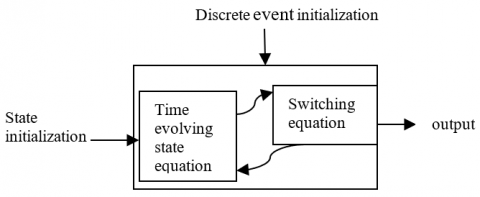Figure 1. Mathematical model of continuous switched system

3. General Structure of the Algorithm of Self-Switched TD-UKF and ML Based Fault Detection

This section has been divided into two sub sections. In the first section the general structure of self-switched estimator has been demonstrated. The second section deals with fault detection using machine learning.

3.1 Estimation and fault detection using time delayed filter

Though the discrete events (i.e. mode) of a NSCS occasionally determined directly from the measurements, but if all measurements are unavailable and especially when the available measurements are time delayed, the estimation becomes a real challenge. The algorithm of Model based FDI using self-switched time delayed estimator is given in Table 1.

Table 1. Algorithm of fault detection using self-switched estimator [8, 9]

 Step 1: Initialize the filter state and error covariance ($\hat{x} ; P_{k}^{-}$) Step 2: Mode Determination based on initialization Step 3: Estimate states using estimator Step 4: Determine modes based on estimated states Step 5: Incorporate the algorithm to estimate the time delay Step 6: Residuals are calculated (Residual is basically the difference of true and estimated value of the state) Step 7: t-statistical tests has been performed and threshold has been determined Step 8: Fault Detection has been done using Machine Learning Method Step 9: Repeat from step 3 for next iteration

3.2 Fault detection using PPCA based SVM

Fault detection and isolation using machine learning is developed in two parts: training of models for FDI and validation of the obtained models. The error statistics derived from the proposed estimator has been taken as input. The detailed algorithm has been discussed in flowchart in Figure 2.

## 2.png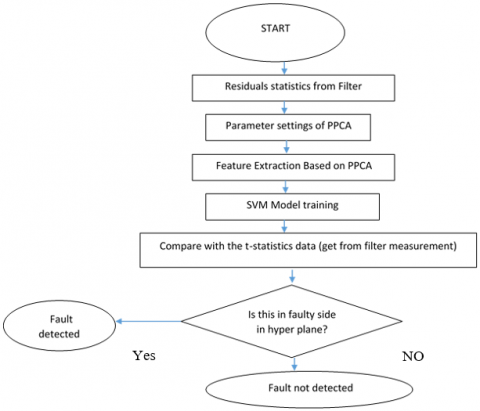Figure 2. Flowchart of fault detection using machine learning

4. Mathematical Formulation of Time Delayed Unscented Kalman Filter

Proposed filter algorithm has given below:

Let, process equation and measurement equation of a plant in discrete domain are given as:

$x_{k}=f_{j}\left(x_{k-1}\right)+w_{k}$       (3)

$y_{k}=h_{j}\left(x_{k}\right)+v_{k}$       (4)

where process noise (wk) and measurement noise (vk) respectively with mean zero and covariances are Q and R respectively. The symbol x(.) denotes the state vector. Let, ykd is the delayed measurement can be defined as:

$y_{k d}=h_{j}\left(x_{k}, l\right)+v_{k, l}$ where $l=k-N ; N$ is the amount of delay.

Filter Algorithm

In this section the details of TD- UKF algorithm has been presented for one single mode. The core algorithm discussed in references [11, 12] has been revisited here.

Let us assume xk is a n X n matrix and yk is the measurement matrix of p X n.

System model can be described as

$x_{k+1}=A x_{k}+process noise.$       (5)

Measurement model can be written as

$y_{k}=C x_{k}+measurement noise$      (6)

Here A is the system matrix and C is the measurement matrix. In this work, both noises are assumed to be Gaussian white noise with covariances Q(k) and R(k) respectively and mean is zero.

Algorithm for Time-delayed UKF(TD-UKF)

Step 1: Initialize the state error covariance (Pk), estimated state (x0), Process noise and measurement noise covariances.

Step 2: Time update (predict as per Kalman Filter equations  to obtain $\dot{\widehat{x_{k}}}$ and $P_{k}^{-}$).

Step 3: Calculate the sigma point.

$\check{x}_{k}=\left[\dot{\widehat{x_{k-1}}}, \dot{\widehat{x_{k-1}}}+\sqrt{(n+\aleph) P_{k}^{-}}, \dot{\widehat{x_{k-1}}}+\sqrt{(n+\aleph) P_{k}^{-}}\right]$       (7)

where, א= a2(n+K)-n.

As the system is nth-order, the least number of sigma points required is n+1 . But, for smoother tracking the number sigma points has been used here are 2n+1=7. The value of ‘a’ has been taken from .

Step 4: update measurement prediction.

$y_{k=}^{-} \sum_{i=0}^{2 n} W i * y k$ Where $W i=\frac{\aleph}{2 \aleph+1}$        (8)

Step 5: The covariance for innovation Pykyk and the cross covariance Pxkyk has been determined as stated in paper .

Step 6: Finally, the Kalman gain is Kk= PxkykP-1ykyk.

Step 7:

$P_{k}=W i\left[\left(\hat{x}_{k}^{-}-\hat{\chi}_{k}^{-}\right)\left(\hat{x}_{k}^{-}-\hat{\chi}_{k}^{-}\right)^{T}\right]$       (9)

$\hat{x}_{k}^{-}=\sum_{i=0}^{2 n} W_{i} * \hat{\chi}_{k}$       (10)

Step 8: Inculcate the time delay.

$M=\prod_{i=0}^{N-1}\left(I-K_{k} * \mathrm{P}_{\mathrm{xkyk}}\right) * P_{y k y k}$       (11)

The estimated state error covariance is given as (where yk is true measurement) .

Step 9: The change due to time delay.

$\delta \hat{x}=M * K *\left(y_{k d}-\hat{y}_{k}^{-}\right)$       (12)

Step 10: State can be updated as:

$\hat{x}_{=} \hat{x}_{1}+\delta \hat{x}$      (13)

Step 11: Covariance can be updated as:

$P_{k+1}=P_{k}-K_{k} * S_{y \hat{x}} * M$      (14)

5. Mathematical Formulation of Probabilistic PCA

Principal Component Analysis (PCA) can not be referred as a predictive analysis tool. Rather, it is basically data pre-processing tool. Here Probabilistic PCA (PPCA) has been used to find the most relevant combination of variables and use them in fault detection model. Here PPCA has also been used to condense the large datasets derived from the t-statistical value, at the same time minimizing information loss. The algorithm is given below.

Algorithm of PPCA for data processing:

 Probabilistic PCA Input: Raw data v, Error rate e Output: $S$, Ufinal, $\bar{v}$ Step 1: Compute the empirical mean shape $\bar{v}=\frac{1}{k} \sum_{j=1}^{k} v_{j}$       (15) Step 2: Compute the co variance matrix C where $C=\frac{1}{K}\left[\left(v_{1}-\bar{v}\right)\left(v_{2}-\bar{v}\right) . .\left(v_{j}-\bar{v}\right) . .\left(v_{k}-\bar{v}\right)\right]{\left[\left(v_{1}-\bar{v}\right)\left(v_{2}-\bar{v}\right) . .\left(v_{j}-\bar{v}\right) . .\left(v_{k}-\bar{v}\right)\right]^{\prime} }$       (16) Step 3: Decompose the Co variance Matrix C=UVDT Step 4: Calculate the Eigen values of D matrix and rearrange it in descending order where ith Eigen value: $\lambda_{i}=d_{i}^{2}(1 \leq i \leq \min (d, k)$       (17) Step 5: for m=1: (d-1) Error E=$\frac{1}{k} \sum_{m=1}^{k}\left\|v_{j}-U_{j} U_{j}{ }^{T}\left(v_{j}-\bar{v}\right)-\bar{v} \cdot\right\|^{2}$       (18) Step 6 Compute S= $\frac{1}{k}=\frac{1}{d-k \sum_{i=k+1}^{d} \quad \lambda_{i}}$        (19) Ufinal $=U_{k} \sqrt{\left(\lambda_{k}-S^{*} I\right)}$       (20)
6. Plant Description

The hybrid nonlinear plant considered in this paper is the well-known three tank system [8, 9]. The specific version of the three tank system addressed in this work is described in the graphic diagram in Figure 3.

## 3.png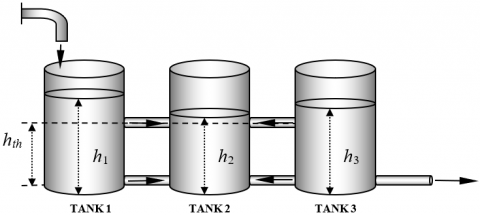Figure 3. Three tank system: Nonlinear switched Continuous system

In this arrangement, when the water column height goes beyond the threshold height as shown in figure, water starts to flow from middle tank to other tanks through the middle connecting pipes. Route of water flow through the bottom connection are governed by on the relative heights of the columns. In this paper the water flow through inlet has been assumed to be constant. The other parameters of three tank system are taken as in paper [8, 10]. As discussed in the aforementioned work, the state equations can be represented as:

$h_{1}(k+1)=h_{1}(k)+T_{s} * \frac{\left(q_{\text {in }}-q_{12 \text { bottom }} \quad - \quad q_{12 \text { middle}} \quad \right)}{A}+w_{1}(k)$       (21)

$h_{2}(k+1)=h_{2}(k)+T_{s} * \frac{\left(q_{12 \text { bottom }} \quad + \quad q_{12 \text { middle }} \quad + \quad q_{23 \text { bottom }} \quad + \quad q_{23 \text { middle }} \quad \right)}{A}+w_{2}(k)$       (22)

$h_{3}(k+1)=h_{3}(k)+T_{s} * \frac{\left(-q_{30}-q_{23 \text { bottom }} \quad - \quad q_{23 \text { middle }} \quad \right)}{A}+w_{3}(k)$       (23)

qin=flow through the inlet of the system.

q30= flow through the outlet of the system.

$a_{30} \cdot s_{30} \cdot \sqrt{2 g h_{3}(k)}$       (24)

qi2bottom= flow through the bottom connecting pipe.

C=measurement matrix. It is assumed that C=[1 1 0].

Output equation: In measurement, it is assumed that the third measurement is absent and other two measurements are having white measurement noises and they are time delayed.

$q_{i 3 b o t t o m}=a_{i 2} \cdot S_{i 2} \cdot \operatorname{sgn}\left(h_{1}(k)\right.\left.-h_{3}(k)\right) \cdot \sqrt{2 g\left|h_{i}(k)-h_{2}(k)\right|}$       (25)

w1, w2, w3=process noises. vp(k) =measurement noises to corresponding measurements.

$q_{i 2 \text { middle }}^{1}=0, h_{1}, h_{2}, h_{3}<h_{t h}$       (26)

$q_{i 2 m i d d l e}^{2}=a_{i 2} s_{i 2} \cdot \sqrt{2 g\left|h_{i}(k)-h_{t h}\right|}, h_{2}<h_{t h}<h_{1,} h_{3}$       (27)

$q_{i 2 \text { middle }}^{3}=-a_{i 2} . S_{i 2} \cdot \sqrt{2 g\left(h_{2}(k)-h_{t h}\right)}, h_{1}, h_{3}<h_{t h}<h_{2}$       (28)

$q_{i 2 \text { middle }}^{4}=a_{i 2} \cdot S_{i 2} \cdot \operatorname{sgn}\left(h_{i}-h_{2}\right) \cdot \sqrt{2 g\left|h_{i}-h_{3}\right|}, h_{1}, h_{2}, h_{3}>h_{v}$        (29)

In this work, two type of faults has been considered to prove the efficacy of the proposed method namely, abrupt fault (sudden change in the flow through inlet pipe) and incipient fault that is clogging between tank 2 and 3 bottom pipe.

Fault case 1:

Clogging between bottom pipe-line of tank 2 and tank 3 has been considered as incipient fault, can be demarcated as:

$a_{23 c l o g}=x^{*} a_{23}$       (30)

where: a23clog = reduced cross-sectional area of the bottom pipe line due to clogging

$\mathrm{x}=\left(1-\delta_{f}\right)$       (31)

a23clog =net cross sectional area under working condition. And δf = percentage of clogged portion.

Fault case 2:

Abrupt fault in this work has been assumed as sudden change in flow through inlet and can be defined as qin= x* qin. Here x defines the flow rate due to abrupt change in the pump flow.

## 4.png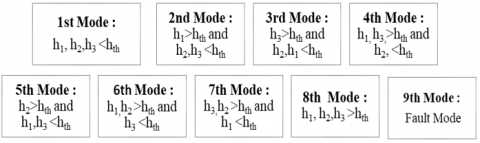Figure 4. Mode discrimination chart

Modes according to the flow rate through the middle connecting pipe can be defined as (Figure 4).

7. Results and Discussion

Case 1: It is assumed a fault free case where the initial condition of each tank has been assumed to be 6 cm. Process noise covariances are taken as 0.1 cm2 and measurement noise covariance has been taken as 1 cm2. From the Figure 5, it can be concluded that normal UKF shows more estimation error than Self switched TD-UKF. It is assumed that 60 second time delay is present in measurement. Mode discrimination is also better in case of TD-UKF.

Case 2: It has been assumed that clogging fault has occurred at 60 sec. The amount of clogging has been taken as 30%. It can be shown from Figure 6 that non delayed UKF cannot at all detect the fault. TD-delayed UKF with t-statistics can detect the fault at 72 sec whereas TD-delayed UKF with ML based FDI algorithm can detect the fault at 68 Sec. Here PPCA has been used for feature extraction of the error data set derived from the estimator.

Case 3: In this case study different types of fault occurrences have been simulated and compared. It can be proved from Table 2 that ML based detection technique gives faster fault detection irrespective of the fault type.

## 5.1.png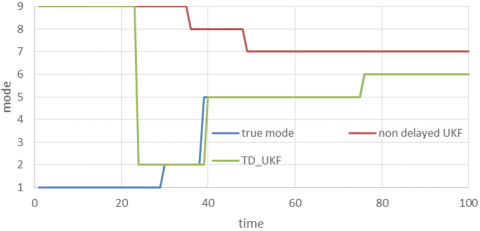(a)

## 5.2.png(b)

Figure 5. (a) Mode discrimination (b) estimation error plot

Table 2. Comparison of different type of fault detection methods

 Type of fault Fault occurrence time (actual) Fault detection using t-statistics Fault detection using ML comment Abrupt fault 200s 210s 205s SVM gives better result Incipient fault 200s 222s 213s SVM gives better result Both fault 200s 225s 214s SVM gives better result

## 6.png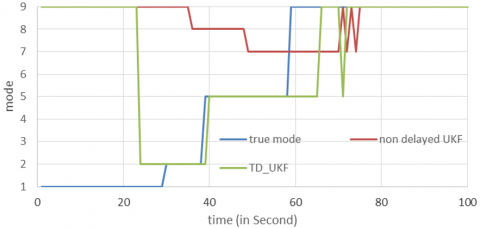Figure 6. Comparison of fault detection using different methods

8. Conclusions

This work characterizes the time delayed UKF based estimation and ML based fault detection techniques for nonlinear switched continuous system (NSCS). These technique employs time delayed UKF successfully for state estimation and switched state (i.e., mode) determination. The characterization is carried out through simulation studies. The research findings of this work are multidimensional. It was evidenced from this work that the proposed time delayed self-switched UKF are able to track the states with satisfactory accuracy and therefore can discriminate the discrete states with less error even though the measurements are time delayed with respect to other estimator paradigm. Secondly, it has been shown that machine learning based fault detection is more accurate and faster than t-statistical based thresholding for FDI. In this paper it is assumed that the noise statistics are known and it is white Gaussian noise. In future, the adaptive estimation for unknown noises or colored noises can be implemented for more accurate and more satisfactory fault detection duties with higher range coverage. Deep learning can also be proposed for such FDI duties in future.

Nomenclature
 h Height of the water column in cm q Water flow rate in cm/sec a Cross sectional area of each pipe in cm2 A Cross sectional area of each tank in cm2 s Flow coefficient through each pipe Subscripts i2bottom Connection of ith water tank with 2nd tank through bottom pipe i2middle Connection of ith water tank with 2nd tank through middle pipe i Tank no:1 or 3
References

 Vachtsevanos, G.J., Vachtsevanos, G.J. (2006). Intelligent fault diagnosis and prognosis for engineering systems. Hoboken: Wiley. ISBN: 978-0-471-72999-0.

 Frank, P.M. (1990). Fault diagnosis in dynamic systems using analytical and knowledge-based redundancy: A survey and some new results. Automatica, 26(3): 459-474. https://doi.org/10.1016/0005-1098(90)90018-D

 Patton, R.J. (1990). Fault detection and diagnosis in aerospace systems using analytical redundancy. Computing & Control Engineering Journal, 2(3): 127-136. https://doi.org/10.1049/cce:19910031

 Buss, M., Glocker, M., Hardt, M., Stryk, O.V., Bulirsch, R., Schmidt, G. (2002). Nonlinear hybrid dynamical systems: modeling, optimal control, and applications. In Modelling, Analysis, and Design of Hybrid Systems, pp. 311-335. https://doi.org/10.1007/3-540-45426-8_18

 Alessandri, A., Coletta, P. (2001). Design of Luenberger observers for a class of hybrid linear systems. In International Workshop on Hybrid Systems: Computation and Control, pp. 7-18. https://doi.org/10.1007/3-540-45351-2_5

 Fazai, R., Abodayeh, K., Mansouri, M., Trabelsi, M., Nounou, H., Nounou, M., Georghiou, G.E. (2019). Machine learning-based statistical testing hypothesis for fault detection in photovoltaic systems. Solar Energy, 190: 405-413. https://doi.org/10.1007/s11633-014-0790-9

 Laouti, N., Othman, S., Alamir, M., Sheibat-Othman, N. (2014). Combination of model-based observer and support vector machines for fault detection of wind turbines. International Journal of Automation and Computing, 11(3): 274-287. https://doi.org/10.1007/s11633-014-0790-9

 Chatterjee, S. (2015). Improved fault detection and identification for nonlinear hybrid systems using self switched CDKF. In 2015 Annual IEEE India Conference (INDICON), pp. 1-6. https://doi.org/10.1109/INDICON.2015.7443175

 Chatterjee, S., Sadhu, S., Ghoshal, T.K. (2017). Improved estimation and fault detection scheme for a class of non-linear hybrid systems using time delayed adaptive CD state estimator. IET Signal Processing, 11(7): 771-779. https://doi.org/10.1049/iet-spr.2016.0380

 Chatterjee, S., Sadhu, S., Ghoshal, T.K. (2015). Fault detection and identification of non-linear hybrid system using self-switched sigma point filter bank. IET Control Theory & Applications, 9(7): 1093-1102. https://doi.org/10.1049/iet-cta.2014.0716

 Sadhu, S., Mondal, S., Srinivasan, M., Ghoshal, T.K. (2006). Sigma point Kalman filter for bearing only tracking. Signal processing, 86(12): 3769-3777. https://doi.org/10.1016/j.sigpro.2006.03.006

 Van Der Merwe, R., Wan, E.A. (2001). The square-root unscented Kalman filter for state and parameter-estimation. In 2001 IEEE international conference on acoustics, speech, and signal processing. Proceedings (Cat. No. 01CH37221), 6: 3461-3464. https://doi.org/10.1109/ICASSP.2001.940586

 Brown, R., Hwang, P. (1992). Introduction to random signals and applied Kalman filtering (Book). New York, John Wiley & Sons, Inc.

 Wang, W., Li, L., Zhou, D., Liu, K. (2007). Robust state estimation and fault diagnosis for uncertain hybrid nonlinear systems. Nonlinear Analysis: Hybrid Systems, 1(1): 2-15. https://doi.org/10.1177/0959651812450113

 Liu, Y., Jiang, J. (2008). Fault diagnosis and prediction of hybrid system based on particle filter algorithm. In 2008 IEEE International Conference on Automation and Logistics, pp. 1491-1495. https://doi.org/10.1109/ICAL.2008.4636389

 Prakash, J., Patwardhan, S.C., Shah, S.L. (2010). State estimation and nonlinear predictive control of autonomous hybrid system using derivative free state estimators. Journal of Process Control, 20(7): 787-799. https://doi.org/10.3182/20131218-3-IN-2045.00090

 Asokan, A., Sivakumar, D. (2007). Fault detection and diagnosis for a three-tank system using structured residual approach. ICGST-ACSE Journal, 7(2): 1-8.

 Noura, H., Theilliol, D., Sauter, D. (2000). Actuator fault-tolerant control design: demonstration on a three-tank-system. International Journal of Systems Science, 31(9): 1143-1155. https://doi.org/10.1080/002077200418414

 Soken, H.E., Sakai, S.I., Wisniewski, R. (2014). In-orbit estimation of time-varying residual magnetic moment. IEEE Transactions on Aerospace and Electronic Systems, 50(4): 3126-3136. https://doi.org/10.1109/TAES.2014.130225

 Alessandri, A., Baglietto, M., Battistelli, G. (2007). Luenberger observers for switching discrete-time linear systems. International Journal of Control, 80(12): 1931-1943. https://doi.org/10.1080/00207170701481683

 Vapnik, V., Golowich, S., Smola, A. (1996). Support vector method for function approximation, regression estimation and signal processing. Advances in Neural Information Processing Systems.

 Chutinan, A., Krogh, B.H. (2003). Computational techniques for hybrid system verification. IEEE Transactions on Automatic Control, 48(1): 64-75. https://doi.org/10.1109/TAC.2002.806655

 Weiss, M., Wiederoder, M.S., Paffenroth, R.C., et al. (2018). Applications of the Kalman filter to chemical sensors for downstream machine learning. IEEE Sensors Journal, 18(13): 5455-5463. https://doi.org/10.1109/JSEN.2018.2836183

 Zou, F., Jiang, X., Wang, T., Lin, Z. (2009). A banyan-tree topology for railway wireless mesh network. In 2009 5th International Conference on Wireless Communications, Networking and Mobile Computing, pp. 1-4. https://doi.org/10.5555/1737966.1738121

 Bemporad, A., Mignone, D., Morari, M. (1999). Moving horizon estimation for hybrid systems and fault detection. In Proceedings of the 1999 American Control Conference (Cat. No. 99CH36251), 4: 2471-2475. https://doi.org/10.1007/978-3-540-31954-2_39

 Naresh Kumar, A., Suchitra, D. (2011). AI based economic load dispatch incorporating wind power penetration. Proceedings of the 2011 International Conference on Electrical Engineering and Informatics, 2011, pp. 1-8. https://doi.org/10.1109/ICEEI.2011.6021520

 Naresh Kumar, A., Sanjay, C., Chakravarthy, M. (2020). Adaptive neuro fuzzy inference system-based fault location technique in double circuit transmission line against simultaneous faults. Multiscale and Multidiscip. Model. Exp. and Des., 3: 143-150. https://doi.org/10.1007/s41939-019-00066-x

 Naresh Kumar, A., Sridhar, P., Anil Kumar, T., Ravi Babu, T., Mohan, V.C.J. (2020). Adaptive neuro-fuzzy inference system based evolving fault locator for double circuit transmission lines. IAES International Journal of Artificial Intelligence, 9(3): 448-455. http://dx.doi.org/10.11591/ijai.v9.i3.pp448-455